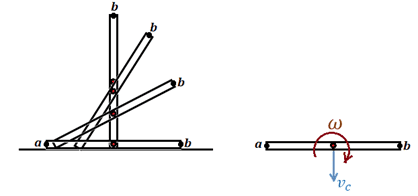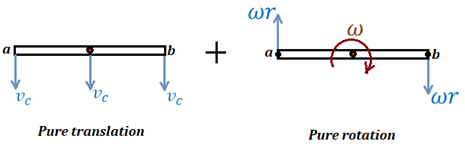# Rotational dynamics and the conservation of energy

• wcjy
It depends whether you think of the coin's motion as the sum of a linearly downward motion and a rotation about its centre, or as just a rotation about its instantaneous centre of rotation (which would be point a).

#### wcjy

Homework Statement
Consider releasing an upright coin from a smooth, frictionless surface. The coin has a radius of 0.01 m and falls sideways. Calculate the tangential velocity of the top of the coin just before the top of the coin hits the surface. You can treat the coin as a uniform disk.
Relevant Equations
I = Icm + md^2
0.5IW^2
mgh
I = Icm + mr^2
I = 0.5 mr^2 + mr^2
I= 3/2 mr^2

By COE,
mgh = 0.5(3/2 mr^2)(w^2)
g(2r) = 3/4(r^2)(w^2)
8g/3 = rw^2 = v^2 / r
v = sqrt( 8gr/3)
v=0.511m/s

ans: v=0.79m/s

•Delta2
wcjy said:
I = Icm + mr^2
What axis will the coin rotate about? Remember, there is no friction.
wcjy said:
I= 3/2 mr^2
What is the moment of inertia of a disc about a diameter?
wcjy said:
g(2r) = 3/4(r^2)(w^2)
How far does the COM descend?

•wcjy and Delta2
haruspex said:
What axis will the coin rotate about? Remember, there is no friction.
it will rotate in the centre of mass

haruspex said:
What is the moment of inertia of a disc about a diameter!
i had a misconception. i thought it was 0.5 mr^2 + mr^2 but after seeing a video i realized it is 0.25mr^2

haruspex said:
How far does the COM descend?
it decends r, i initially thought it counts from the top -.- sry

im still confused how to solve it.
since it is a frictionless surface, the coin will rotate around its cm. so inertia is 0.5mr^2? but when it rotate, the coin will also fall, causing the cm to change?

What makes that coin rotate?
Could you post a diagram?

wcjy said:
the coin will rotate around its cm. so inertia is 0.5mr^2?
Yes, it is rotating about its cm, but the axis is a diameter. As you posted, that makes it mr2/4.
wcjy said:
the coin will also fall, causing the cm to change?
The position of the cm in space changes, but the coin continues to rotate about its cm wherever that is.

•wcjy
so can i say that mgh =0.5mv'^2 (velocity of cm moving down) + 0.5(mr^2/4)w^2
and v'^2 cannot be used in the w cause they are two different entities
cause v' is the velocity of cm moving down while the v in w is the tangential velocity

wcjy said:
so can i say that mgh =0.5mv'^2 (velocity of cm moving down) + 0.5(mr^2/4)w^2
and v'^2 cannot be used in the w cause they are two different entities
cause v' is the velocity of cm moving down while the v in w is the tangential velocity
Yes, but you can assume the coin maintains contact with the ground. That gives a relationship between the linear and angular velocities.

•wcjy
mgh = mv^2 /2 + 0.5(mr^2 / 4)w^2
gh = r^2w^2 /2 + r^2w^2 /8
gh = (r^2w^2)(1/5 + 1/8)
gh = (v^2)(0.625)
v = sqrt( 9.8 *0.01 /0.625)
v = 0.395m/s

wcjy said:
mgh = mv^2 /2 + 0.5(mr^2 / 4)w^2
gh = r^2w^2 /2 + r^2w^2 /8
I didn't say what the relationship is between v and w, I left you to work that out.
This is not a rolling disc.

•wcjy
oh so i must use angular momentum

wcjy said:
oh so i must use angular momentum
No, it's just kinematics.

wcjy said:
v = 0.395m/s
v represents the speed of what point of the coin?

erm so there is two types of v. v' = velocity of the cm of the coin and v = tangential velocity of coin.
currently, I am thinking of a way to link them together.
so i trying to link them via time

for rotational part,
time taken for coin from upright position to horizontal position = pi/2w

for translational part,
vt=r

combining them is
v = 2wr/pi

Last edited:
wcjy said:
mgh = mv^2 /2 + 0.5(mr^2 / 4)w^2
gh = r^2w^2 /2 + r^2w^2 /8
gh = (r^2w^2)(1/5 + 1/8)
gh = (v^2)(0.625)
v = sqrt( 9.8 *0.01 /0.625)
v = 0.395m/s
Sorry, I forgot you are only interested in the velocity when the coin lands. I was thinking about the relationship between v and w at an arbitrary point.
Yes, v=wr at that point if v is the linear velocity of the mass centre, but as @TSny hints, that is not the velocity you are asked to find.

TSny said:
v represents the speed of what point of the coin?
translational velocity of coin just before it hits the ground.Let ##b## be the top point. Just as the coin reaches the horizontal postition, what is the velocity of point ##a##? Use this fact to deduce ##\omega## in terms of ##v_c## at this instant. Then think about the velocity of ##b## in terms of ##v_c##.

•Lnewqban
TSny said:
View attachment 270383

Let ##b## be the top point. Just as the coin reaches the horizontal postition, what is the velocity of point ##a##? Use this fact to deduce ##\omega## in terms of ##v_c## at this instant. Then think about the velocity of ##b## in terms of ##v_c##.
sorry I am having a hard time understanding the first part of your sentence. isn't the velocity of point a the tangential velocity? which is the same for both a and b.

wcjy said:
sorry I am having a hard time understanding the first part of your sentence. isn't the velocity of point a the tangential velocity? which is the same for both a and b.
It depends whether you think of the coin's motion as the sum of a linearly downward motion and a rotation about its centre, or as just a rotation about its instantaneous centre of rotation (which would be point a).
Try it this way:
At the instant before impact, in terms of r and ω, what are the vertical velocities of i) point a, ii) the centre of the coin, and iii) point b?

wcjy said:
sorry I am having a hard time understanding the first part of your sentence. isn't the velocity of point a the tangential velocity? which is the same for both a and b.
Relative to the center of mass, points ##a## and ##b## move in circular motion and have the same tangential speed ##\omega r##. But, relative to the laboratory reference frame, the two points have different speeds. In fact, can you see that point ##a## is essentially at rest relative to the surface just before the coin strikes the surface?

give me a few minutes to think about it. at the moment can't see it

TSny said:
Relative to the center of mass, points ##a## and ##b## move in circular motion and have the same tangential speed ##\omega r##. But, relative to the laboratory reference frame, the two points have different speeds. In fact, can you see that point ##a## is essentially at rest relative to the surface just before the coin strikes the surface?
i saw an animation from WolframAlpha. i think i am kind of able to visualise but i wouldn't say that I am certain

Let ##\vec v_a## and ##\vec v_c## be the velocity of ##a## and the velocity of the center of mass, ##c##, relative to the laboratory. Let ##\vec v_{a/c}## be the velocity of ##a## relative to the center of mass. Using the concept of relative velocity we have, $$\vec v_a = \vec v_c + \vec v_{a/c}$$
Does either ##\vec v_c## or ##\vec v_{a/c}## have a non-zero horizontal component just before the coin strikes the table?

no

wcjy said:
no
Ok. So, the relative velocity formula ##\vec v_a = \vec v_c + \vec v_{a/c}## implies that point ##a## has zero horizontal velocity just before the coin strikes the table. Also, since point ##a## stays on the surface, it never has a vertical component of velocity.

ok.. but can you help me understand how does this link to finding Vc in terms of W

wcjy said:
ok.. but can you help me understand how does this link to finding Vc in terms of W
Since ##\vec v_a = 0## just before the coin strikes the table, the relative velocity formula implies that ##\vec v_c + \vec v_{a/c} = 0## at this instant. This will tell you how ##\omega## is related to ##v_c## if you can see how to express ##v_{a/c}## in terms of ##\omega## and ##r##.

•wcjy
TSny said:
Since ##\vec v_a = 0## just before the coin strikes the table, the relative velocity formula implies that ##\vec v_c + \vec v_{a/c} = 0## at this instant. This will tell you how ##\omega## is related to ##v_c## if you can see how to express ##v_{a/c}## in terms of ##\omega## and ##r##.

the way i see it is that if the velocity of a is supposed to be rw, then for it to seem like it is '0' from the center, then Va/c should be = -rw.
this is probably wrong. but this is how i see it. i think my understanding of relative velocity is still not there

Pictorially, you can think of the motion of the coin as a combination of pure translation plus pure rotation about the center of mass. Just before the coin strikes the surface, the picture would be as shown belowThe net velocity of point ##a## is ##v_c## downward plus ##\omega r## upward. These must combine to give zero net velocity for point ##a##.

•Lnewqban and wcjy
ok i think i get it how to solve the problem but can you give me time to think of questions to ask regarding this topic? i wouldn't say i 100% grasp this topic.
thanks so much for helping me.

Ok, sounds good.